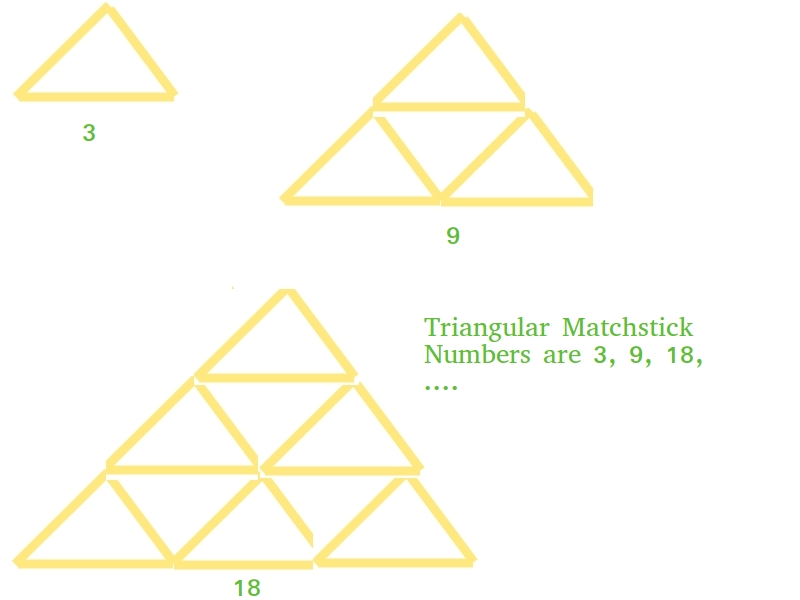Open in App
Not now

# Triangular Matchstick Number

• Difficulty Level : Easy
• Last Updated : 22 Jun, 2022

Given a number X which represents the floor of a matchstick pyramid, write a program to print the total number of matchstick required to form pyramid of matchsticks of x floors.

Examples:

```Input : X = 1
Output : 3

Input : X = 2
Output : 9 ```

This is mainly an extension of triangular numbers. For a number X, the matchstick required will be three times of X-th triangular numbers, i.e., (3*X*(X+1))/2## C++

 `// C++ program to find X-th triangular` `// matchstick number`   `#include ` `using` `namespace` `std;`   `int` `numberOfSticks(``int` `x)` `{` `    ``return` `(3 * x * (x + 1)) / 2;` `}`   `int` `main() ` `{` `    ``cout<

## Java

 `// Java program to find X-th triangular` `// matchstick number` `public` `class` `TriangularPyramidNumber {` `    ``public` `static` `int` `numberOfSticks(``int` `x)` `    ``{` `        ``return` `(``3` `* x * (x + ``1``)) / ``2``;` `    ``}` `    ``public` `static` `void` `main(String[] args)` `    ``{` `        ``System.out.println(numberOfSticks(``7``));` `    ``}` `}`

## Python3

 `# Python program to find X-th triangular` `# matchstick number`   `def` `numberOfSticks(x):` `    ``return` `(``3` `*` `x ``*` `(x ``+` `1``)) ``/` `2` `    `  `# main()` `print``(``int``(numberOfSticks(``7``)))`

## C#

 `// C# program to find X-th triangular` `// matchstick number` `using` `System;`   `class` `GFG` `{` `    ``// Function to ind missing number` `    ``static` `int` `numberOfSticks(``int` `x)` `    ``{` `        ``return` `(3 * x * (x + 1)) / 2;` `    ``}`   `    ``public` `static` `void` `Main()` `    ``{` `        ``Console.Write(numberOfSticks(7));` `    ``}` `}`   `// This code is contributed by _omg`

## PHP

 ``

## Javascript

 ``

Output:

`84`

Time Complexity: O(1)

Auxiliary Space: O(1)

My Personal Notes arrow_drop_up
Related Articles